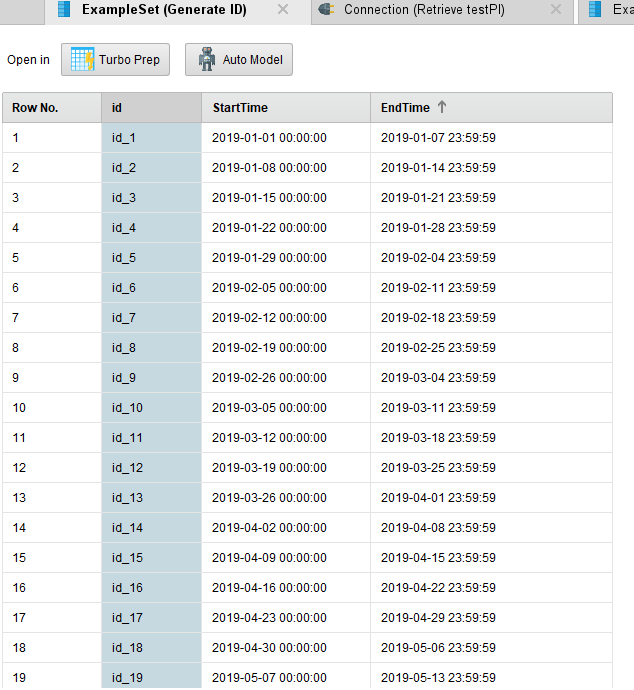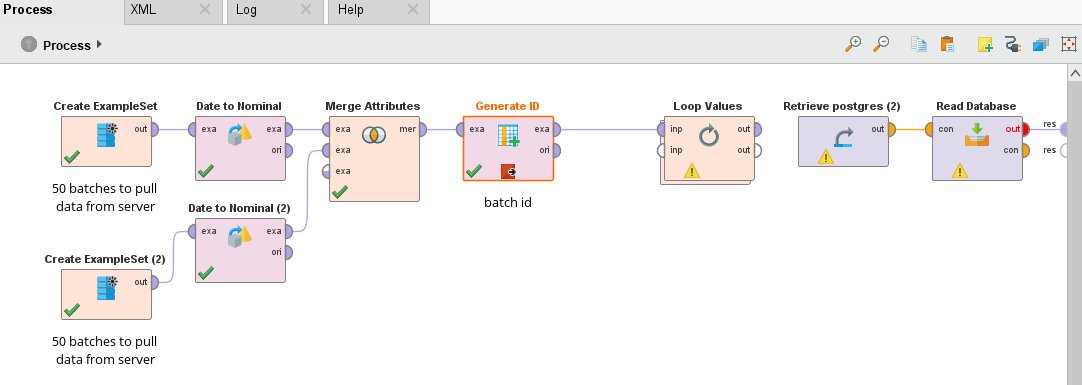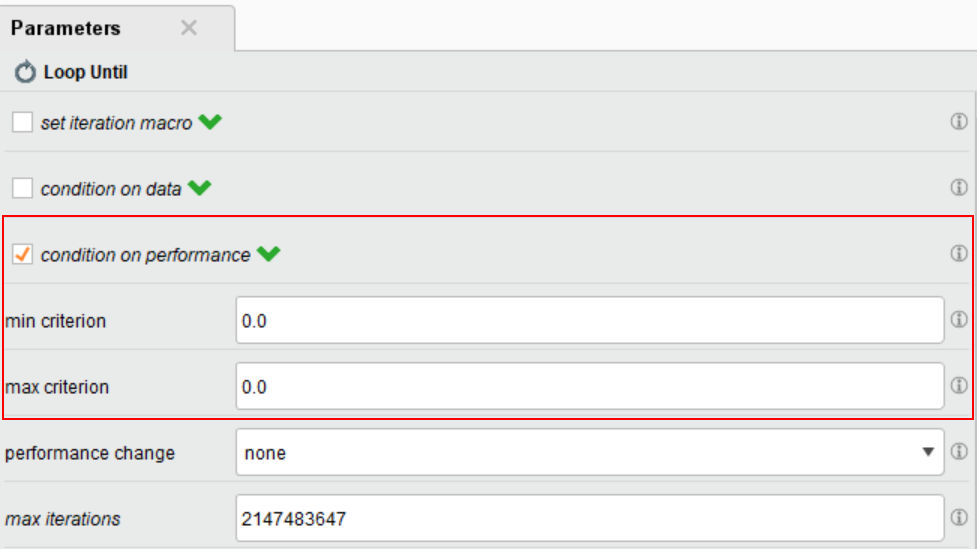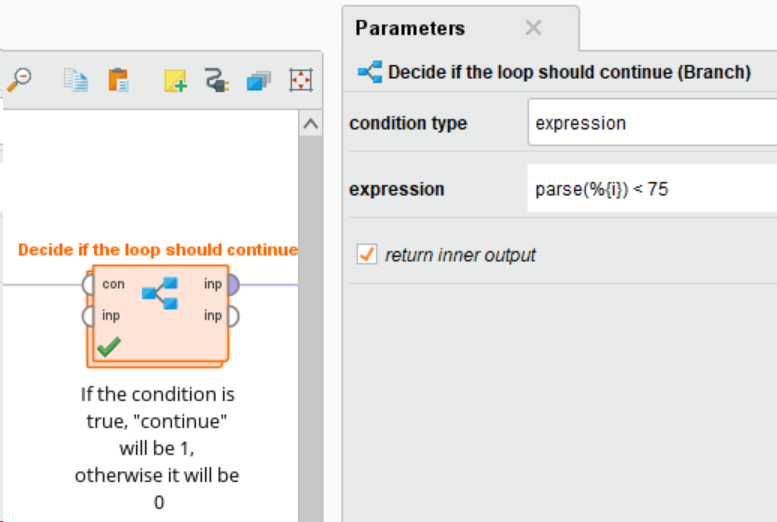# Loop until the value of two macros are equal?

Member Posts: 11Contributor II
edited March 2020 in Help
Hello, I am looking to pull a large amount of data from a server which limits how many points i can pull each time i access it. I would like to get around this by looping the pull and building the table iteratively.

Below is some pseudo code of what i'd like to do:

INSIDE LOOP
%{Moving End} = IF %{Start Time} + %{Step Size} < %{Final End}
THEN %{Start Time} + %{Step Size}
ELSE %{Final End}
Execute Data Pull Block
Append New Pull to Table
%{Start Time} = %{Moving End}

BREAK IF %{Start Time}=%{Final End}

Any help on how to tell the loop to break on this condition would be appreciated, thanks!.
Tagged:

• Administrator, Employee, RapidMiner Certified Analyst, RapidMiner Certified Expert, Member Posts: 363RM Data Scientist
Hi Zak,

Are you trying to pull data  in batches from OSI server (cc. @Michael )? You can list the timestamps for start time and end time in a reference table and apply "loop values" with macro.Let me know if you have followup questions..

Cheers,
YY
• Member Posts: 11Contributor II
Yeah, I am trying to pull from the OSI server. I'll give this a shot tomorrow morning before we speak and see how it works, thanks!
• Administrator, Employee, RapidMiner Certified Analyst, RapidMiner Certified Expert, Member Posts: 31RM Data Scientist
Hi Zak,
We have been discussing adding support for client-side batching internally, but have so far not given it high priority. Reason being that we are simply not sure how often users would run into the limits given that the PI System allows to do common pre-processing steps on the server side (e.g., creating compact equidistant time series).
Do you consider pulling more data than the server allows in a single request a common scenario?
Best,
Michael
• Member Posts: 11Contributor II
So a medium standard data dig for one of our engineers i'd estimate to be like 25 tags, 1 hour resolution over a year = 219,000 data points which exceeds the 150,000 max. I am working on bigger pulls so mine are in the realm of 50 - 100 million data points. From my view it would be common.

As a side note I have figured out how to automatically add in a tag list from excel using the "Set Macros from Example Set" and it works well so that ask can fall down the list a bit.
• Administrator, Employee, RapidMiner Certified Analyst, RapidMiner Certified Expert, Member Posts: 31RM Data Scientist
Thanks for the insights. Guess we have to revisit the client-side batching.
The side note is interesting, too. A simple way to provide similar functionality without having to use macros might be to add an optional input that if connected replaces the data item parameters (just as the connection input replaces the connection parameter).
• Member Posts: 50Guru
edited March 2021
I also had a need to Loop until some condition was reached, but could not find how to directly use the Loop Until operator. Here is the solution I found using some creative abuse of the built-in operators. Since the operator wants a Performance object to decide if the loop should continue, I first create an example set with a boolean variable "continue" based on the result of an expression, then extract that variable using the "Extract Performance" operator configured with optimization direction = minimize. The Loop Until operator is also configured to require a performance between 0 and 0, so it will keep looping while the dummy performance metric (continue) is 1, then stop when it reaches 0.My sample process actually knows in advance how many iterations are needed, as the loop condition is "parse(%{i}) < 75", so a Loop Until is not really necessary in this case. However, the same solution can be used when the number of iterations isn't known in advance.

It would be interesting for future versions to have a new option in the "Loop Until" operator to loop until a user-specified expression becomes true, which is what I would really expect from an operator named "Loop Until". Also, the operator needs some documentation and samples, how to use it is really not clear and I had to find out mostly by trial and error.

`<?xml version="1.0" encoding="UTF-8"?><process version="9.8.001">  <context>    <input/>    <output/>    <macros>      <macro>        <key>i</key>        <value>0</value>      </macro>    </macros>  </context>  <operator activated="true" class="process" compatibility="9.8.001" expanded="true" name="Process">    <parameter key="logverbosity" value="init"/>    <parameter key="random_seed" value="2001"/>    <parameter key="send_mail" value="never"/>    <parameter key="notification_email" value=""/>    <parameter key="process_duration_for_mail" value="30"/>    <parameter key="encoding" value="SYSTEM"/>    <process expanded="true">      <operator activated="true" class="loop_until" compatibility="9.8.001" expanded="true" height="82" name="Loop Until" width="90" x="246" y="85">        <parameter key="set_iteration_macro" value="false"/>        <parameter key="macro_name" value="iteration"/>        <parameter key="macro_start_value" value="1"/>        <parameter key="condition_on_data" value="false"/>        <parameter key="min_attributes" value="0"/>        <parameter key="max_attributes" value="0"/>        <parameter key="min_examples" value="0"/>        <parameter key="max_examples" value="2147483647"/>        <parameter key="condition_on_performance" value="true"/>        <parameter key="min_criterion" value="0.0"/>        <parameter key="max_criterion" value="0.0"/>        <parameter key="performance_change" value="none"/>        <parameter key="max_iterations" value="2147483647"/>        <parameter key="limit_time" value="false"/>        <parameter key="timeout" value="1"/>        <parameter key="condition_before" value="false"/>        <process expanded="true">          <operator activated="true" class="generate_macro" compatibility="9.8.001" expanded="true" height="82" name="Generate Macro" width="90" x="179" y="85">            <list key="function_descriptions">              <parameter key="i" value="parse(%{i})+1"/>            </list>          </operator>          <operator activated="true" class="branch" compatibility="9.8.001" expanded="true" height="82" name="Decide if the loop should continue" width="90" x="313" y="85">            <parameter key="condition_type" value="expression"/>            <parameter key="condition_value" value="parse(i) &lt; 1000"/>            <parameter key="expression" value="parse(%{i}) &lt; 75"/>            <parameter key="io_object" value="ANOVAMatrix"/>            <parameter key="return_inner_output" value="true"/>            <process expanded="true">              <operator activated="true" class="utility:create_exampleset" compatibility="9.8.001" expanded="true" height="68" name="Create ExampleSet" width="90" x="246" y="34">                <parameter key="generator_type" value="comma separated text"/>                <parameter key="number_of_examples" value="100"/>                <parameter key="use_stepsize" value="false"/>                <list key="function_descriptions"/>                <parameter key="add_id_attribute" value="false"/>                <list key="numeric_series_configuration"/>                <list key="date_series_configuration"/>                <list key="date_series_configuration (interval)"/>                <parameter key="date_format" value="yyyy-MM-dd HH:mm:ss"/>                <parameter key="time_zone" value="SYSTEM"/>                <parameter key="input_csv_text" value="iteration,continue&#10;%{i},1"/>                <parameter key="column_separator" value=","/>                <parameter key="parse_all_as_nominal" value="false"/>                <parameter key="decimal_point_character" value="."/>                <parameter key="trim_attribute_names" value="true"/>                <description align="center" color="transparent" colored="false" width="126">Expression is true, set Continue to 1</description>              </operator>              <connect from_op="Create ExampleSet" from_port="output" to_port="input 1"/>              <portSpacing port="source_condition" spacing="0"/>              <portSpacing port="source_input 1" spacing="0"/>              <portSpacing port="sink_input 1" spacing="0"/>              <portSpacing port="sink_input 2" spacing="0"/>            </process>            <process expanded="true">              <operator activated="true" class="utility:create_exampleset" compatibility="9.8.001" expanded="true" height="68" name="Create ExampleSet (2)" width="90" x="179" y="34">                <parameter key="generator_type" value="comma separated text"/>                <parameter key="number_of_examples" value="100"/>                <parameter key="use_stepsize" value="false"/>                <list key="function_descriptions"/>                <parameter key="add_id_attribute" value="false"/>                <list key="numeric_series_configuration"/>                <list key="date_series_configuration"/>                <list key="date_series_configuration (interval)"/>                <parameter key="date_format" value="yyyy-MM-dd HH:mm:ss"/>                <parameter key="time_zone" value="SYSTEM"/>                <parameter key="input_csv_text" value="iteration,continue&#10;%{i},0"/>                <parameter key="column_separator" value=","/>                <parameter key="parse_all_as_nominal" value="false"/>                <parameter key="decimal_point_character" value="."/>                <parameter key="trim_attribute_names" value="true"/>                <description align="center" color="transparent" colored="false" width="126">Expression is false, set Continue to 0</description>              </operator>              <connect from_op="Create ExampleSet (2)" from_port="output" to_port="input 1"/>              <portSpacing port="source_condition" spacing="0"/>              <portSpacing port="source_input 1" spacing="0"/>              <portSpacing port="sink_input 1" spacing="0"/>              <portSpacing port="sink_input 2" spacing="0"/>            </process>            <description align="center" color="transparent" colored="false" width="126">If the condition is true, &amp;quot;continue&amp;quot; will be 1, otherwise it will be 0</description>          </operator>          <operator activated="true" class="extract_performance" compatibility="9.8.001" expanded="true" height="82" name="Use continue as a dummy performance metric" width="90" x="581" y="85">            <parameter key="performance_type" value="data_value"/>            <parameter key="statistics" value="average"/>            <parameter key="attribute_name" value="continue"/>            <parameter key="example_index" value="1"/>            <parameter key="optimization_direction" value="minimize"/>            <description align="center" color="transparent" colored="false" width="126">The loop is handled as an optimization problem where the objective is to minimize the &amp;quot;continue&amp;quot; variable until it reaches 0 or less</description>          </operator>          <operator activated="true" class="multiply" compatibility="9.8.001" expanded="true" height="103" name="Multiply" width="90" x="782" y="136">            <description align="center" color="transparent" colored="false" width="126">Provide all outputs the Loop Until operator wants even if we don't use them</description>          </operator>          <connect from_op="Generate Macro" from_port="through 1" to_op="Decide if the loop should continue" to_port="condition"/>          <connect from_op="Decide if the loop should continue" from_port="input 1" to_op="Use continue as a dummy performance metric" to_port="example set"/>          <connect from_op="Use continue as a dummy performance metric" from_port="performance" to_port="performance"/>          <connect from_op="Use continue as a dummy performance metric" from_port="example set" to_op="Multiply" to_port="input"/>          <connect from_op="Multiply" from_port="output 1" to_port="example set"/>          <connect from_op="Multiply" from_port="output 2" to_port="output 1"/>          <portSpacing port="source_input 1" spacing="0"/>          <portSpacing port="sink_performance" spacing="0"/>          <portSpacing port="sink_example set" spacing="84"/>          <portSpacing port="sink_output 1" spacing="0"/>          <portSpacing port="sink_output 2" spacing="0"/>        </process>      </operator>      <operator activated="true" class="append" compatibility="9.8.001" expanded="true" height="82" name="Append" width="90" x="380" y="85">        <parameter key="datamanagement" value="double_array"/>        <parameter key="data_management" value="auto"/>        <parameter key="merge_type" value="all"/>      </operator>      <connect from_op="Loop Until" from_port="output 1" to_op="Append" to_port="example set 1"/>      <connect from_op="Append" from_port="merged set" to_port="result 1"/>      <portSpacing port="source_input 1" spacing="0"/>      <portSpacing port="sink_result 1" spacing="0"/>      <portSpacing port="sink_result 2" spacing="0"/>    </process>  </operator></process>`